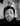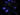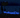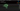Navigate back to the homepage

# Want to make a cool shader in three ? then come read on!Rick
March 27th, 2023 · 1 min readI’m a big advacate of learning by doing something. In my spare time around my day job I practice shaders quite often; I find it very useful to play around with node graphs in software like blender, unity or unreal. The benefit being you get a deep understanding of the code behind the nodes, which allow you to do more complex and exciting shaders.

This was a project which was really fun to do! and I did around christmass 🙂

It involved playing around with noise and applying this to on hover or onEnter event in @react-three/fiber.

Please find the code below, this involved a pretty basic vertex and fragment shader.

```.css-1chxjt6{position:absolute;right:22px;top:24px;padding:8px 12px 7px;border-radius:5px;color:#6f7177;-webkit-transition:background 0.3s ease;transition:background 0.3s ease;}.css-1chxjt6:hover{background:rgba(255,255,255,0.07);}.css-1chxjt6[data-a11y="true"]:focus::after{content:"";position:absolute;left:-2%;top:-2%;width:104%;height:104%;border:2px solid var(--theme-ui-colors-accent,#6166DC);border-radius:5px;background:rgba(255,255,255,0.01);}@media (max-width:45.9375em){.css-1chxjt6{display:none;}}1varying vec2 vUv;2varying vec3 pos;3varying vec3 norm;4
5void main() {6  vUv = uv;7  norm = normal;8  pos = (vec4(position, 1.0)).xyz;9  gl_Position = projectionMatrix * modelViewMatrix * vec4(position, 1.0);10}```
```1uniform float time;2uniform vec2 resolution;3uniform vec3 origin;4uniform float radius;5varying vec2 vUv;6varying vec3 pos;7
8
9#pragma glslify: random = require(glsl-random)10#define iterations 2511#define mod3 vec3(.1031, .11369, .13787)12
13vec3 hash3_3(vec3 p3) {14p3 = fract(p3 * mod3);15  p3 += dot(p3, p3.yxz + 19.19);16  return -1. + 2. * fract(vec3((p3.x + p3.y) * p3.z, (p3.x+p3.z) * p3.y, (p3.y+p3.z) * p3.x));17}18
19float perlin_noise3(vec3 p) {20  vec3 pi = floor(p);21  vec3 pf = p - pi;22
23  vec3 w = pf * pf * (3. - 2. * pf);24
25  return    mix(26    mix(27          mix(28              dot(pf - vec3(0, 0, 0), hash3_3(pi + vec3(0, 0, 0))),29              dot(pf - vec3(1, 0, 0), hash3_3(pi + vec3(1, 0, 0))),30              w.x),31          mix(32              dot(pf - vec3(0, 0, 1), hash3_3(pi + vec3(0, 0, 1))),33              dot(pf - vec3(1, 0, 1), hash3_3(pi + vec3(1, 0, 1))),34              w.x),35    w.z),36      mix(37          mix(38              dot(pf - vec3(0, 1, 0), hash3_3(pi + vec3(0, 1, 0))),39              dot(pf - vec3(1, 1, 0), hash3_3(pi + vec3(1, 1, 0))),40              w.x),41          mix(42              dot(pf - vec3(0, 1, 1), hash3_3(pi + vec3(0, 1, 1))),43              dot(pf - vec3(1, 1, 1), hash3_3(pi + vec3(1, 1, 1))),44              w.x),45     w.z),46w.y);47}48
49
50float noise_sum_abs3(vec3 p) {51  float f = 0.;52  p = p * 3.;53  f += 1.0000 * abs(perlin_noise3(p)); p = 2. * p;54  f += 0.5000 * abs(perlin_noise3(p)); p = 3. * p;55  f += 0.2500 * abs(perlin_noise3(p)); p = 4. * p;56  f += 0.1250 * abs(perlin_noise3(p)); p = 5. * p;57  f += 0.0625 * abs(perlin_noise3(p)); p = 6. * p;58
59  return f;60}61
62
63
64void main() {65  vec2 temp = vUv;66  float noise = noise_sum_abs3(pos * 20.2 / radius * 0.1);67  vec3 mixed = mix(pos, origin, noise);68
69  vec3 color = vec3(0.01* (noise), 0.01*  (noise), 0.02 *  (noise));70
71  float noisyRadius = radius * (noise);72  if (noisyRadius - length(pos - origin) > 0.0) {73    if (noisyRadius - length(pos - origin) < pow(noise, 9.0) * 50.0) {74      color = vec3(0.0021 * noise * length(mixed) * 40.0 / length(pos - origin) ,0.0021 * noise * length(mixed) * 40.0 / length(pos - origin),0.05 * noise * length(mixed) * 40.0 / length(pos - origin));75    }76  }77  gl_FragColor.rgba = vec4(color, 1.0);78}```

The vertex shader is just bog standard with nothing special but several varyings we pass to the fragment shader.

The fragment shader is where the magic happens and is where the visuals come from.

The uniforms then varyings go first as usual in the fragment shader.We have some noise functions at the top of the file which we use to shape the surface effect on this cube.

```1// Get noise value using expanding or contracting radius2float noise = noise_sum_abs3(pos * 20.2 / radius * 0.1);3
4// We get a point between the position of the fragment and the origin5// of the hover effect. This essentially maps the shape of the noise // onto the color below.6vec3 mixed = mix(pos, origin, noise);7
8// This is the whiter color which gives the greyed out effect.9vec3 color = vec3(0.01* (noise), 0.01*  (noise), 0.02 *  (noise));10
11float noisyRadius = radius * (noise);12if (noisyRadius - length(pos - origin) > 0.0) {13  if (noisyRadius - length(pos - origin) < pow(noise, 9.0) * 50.0) {14    // This is the part of the code which gives the white and blue colors15    color = vec3(0.0021 * noise * length(mixed) * 40.0 / length(pos - origin) ,0.0021 * noise * length(mixed) * 40.0 / length(pos - origin),0.05 * noise * length(mixed) * 40.0 / length(pos - origin));16  }17}18
19// So we either have the greayed out colours or the whitey blue colors.20gl_FragColor.rgba = vec4(color, 1.0);```

Above is a brief explanation of what the code does, alot of this was me playing around with gradiented noise and SDF’s/noise radi.

Hope you like playing around with it.

Pretty short article but have a play with the code and add or remove things!

### More articles from theFrontDev### How to create a immersive 3D environment for the web

The number 1 walk-through for creating an immersive environment for the web. A in depth look on converting a blender project into a optimized web project. Including amazing fireflies, grass, and HDRI image creation.

January 14th, 2023 · 7 min read### Creating An Amazing Particle Transition In Blender and Exporting to React Three Fiber

Highlighting a workflow from blender to React Three Fiber (R3F) for morphing particles from different shapes. Utilizing images for storing data in blender and then consuming in a React Three Fiber / Threejs shader.

November 27th, 2022 · 3 min read